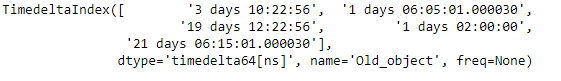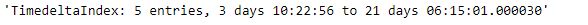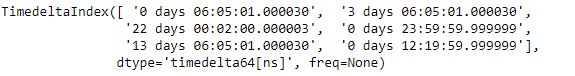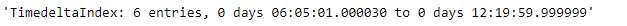Related Articles
Python | Pandas TimedeltaIndex.summary()
• Last Updated : 06 Jan, 2019

Python is a great language for doing data analysis, primarily because of the fantastic ecosystem of data-centric python packages. Pandas is one of those packages and makes importing and analyzing data much easier.

Pandas` TimedeltaIndex.summary()` function return a summarized representation of the given TimedeltaIndex object. The summary contains the total number of entries, first entry and the last entry

Syntax : TimedeltaIndex.summary(value = None)

Parameters :
value : Scalar value to use to fill holes (e.g. 0). This value cannot be a list-likes.

Returns : Summary

Example #1: Use `TimedeltaIndex.summary()` function to find the summary of the given TimedeltaIndex object.

 `# importing pandas as pd``import` `pandas as pd`` ` `# Create the TimedeltaIndex object``tidx ``=` `pd.TimedeltaIndex(data ``=``[``'3 days 10:22:56'``, ``'1 days 06:05:01.000030'``,``                        ``'19 days 12:22:56'``,``'1 days 02:00:00'``, ``                        ``'21 days 06:15:01.000030'``],name ``=``'Old_object'``)`` ` `# Print the TimedeltaIndex object``print``(tidx)`

Output :Now we will use the `TimedeltaIndex.summary()` function to return the summary of the tidx object.

 `# find summary of tidx``tidx.summary()`

Output :As we can see in the output, the `TimedeltaIndex.summary()` function has returned a complete summary of the given TimedeltaIndex object.

Example #2: Use `TimedeltaIndex.summary()` function to find the summary of the given TimedeltaIndex object.

 `# importing pandas as pd``import` `pandas as pd`` ` `# Create the TimedeltaIndex object``tidx ``=` `pd.TimedeltaIndex(data ``=``[``'06:05:01.000030'``, ``'3 days 06:05:01.000030'``, ``                        ``'22 day 2 min 3us 10ns'``, ``'+23:59:59.999999'``,``                        ``'13 days 06:05:01.000030'``, ``'+12:19:59.999999'``])`` ` `# Print the TimedeltaIndex object``print``(tidx)`

Output :Now we will use the `TimedeltaIndex.summary()` function to return the summary of the tidx object.

 `# find summary of tidx``tidx.summary()`

Output :As we can see in the output, the `TimedeltaIndex.summary()` function has returned a complete summary of the given TimedeltaIndex object.

Attention geek! Strengthen your foundations with the Python Programming Foundation Course and learn the basics.

To begin with, your interview preparations Enhance your Data Structures concepts with the Python DS Course.

My Personal Notes arrow_drop_up